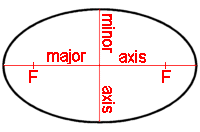## Ellipse Calculator

 major axis = units minor axis = units eccentricity = area = square units foci distance = units loop length = units *perimeter* = units
significant figures

Ellipses have two mutually perpendicular axes about which the ellipse is symmetric. These axes intersect at the center of the ellipse due to this symmetry. The larger of these two axes, which corresponds to the largest distance between antipodal points on the ellipse, is called the major axis or transverse diameter.The calculation is made easier here.

Thinkcalculator.com provides you helpful and handy calculator resources.